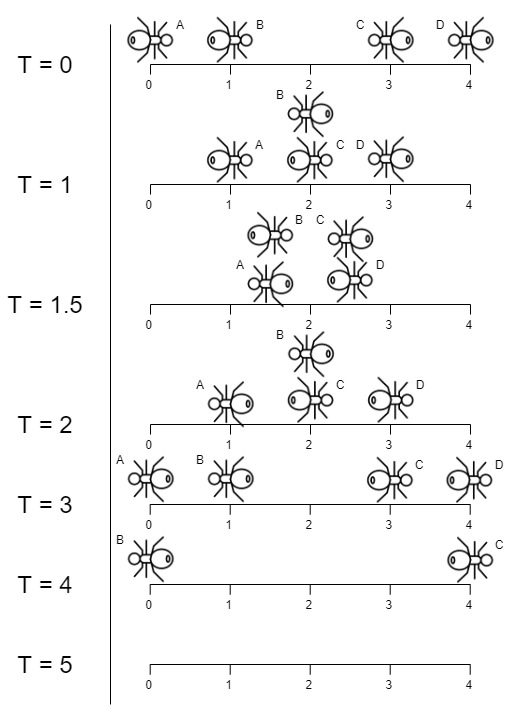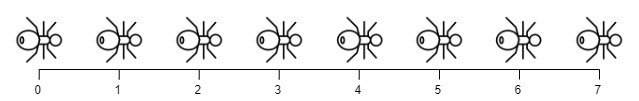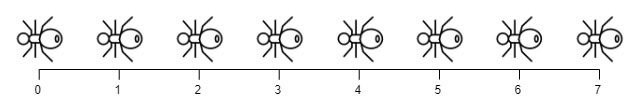# 所有蚂蚁掉下来前的最后一刻

## 1503. 所有蚂蚁掉下来前的最后一刻 (Medium)```输入：n = 4, left = [4,3], right = [0,1]

-下标 0 处的蚂蚁命名为 A 并向右移动。
-下标 1 处的蚂蚁命名为 B 并向右移动。
-下标 3 处的蚂蚁命名为 C 并向左移动。
-下标 4 处的蚂蚁命名为 D 并向左移动。```输入：n = 7, left = [], right = [0,1,2,3,4,5,6,7]

``````输入：n = 7, left = [0,1,2,3,4,5,6,7], right = []

```

```输入：n = 9, left = , right = 

```

```输入：n = 6, left = , right = 

```

• `1 <= n <= 10^4`
• `0 <= left.length <= n + 1`
• `0 <= left[i] <= n`
• `0 <= right.length <= n + 1`
• `0 <= right[i] <= n`
• `1 <= left.length + right.length <= n + 1`
• `left``right` 中的所有值都是唯一的，并且每个值 只能出现在二者之一 中。

[脑筋急转弯] [数组]